# 1 Linear Equations in Linear Algebra LINEAR INDEPENDENCE

• Slides: 321 Linear Equations in Linear Algebra LINEAR INDEPENDENCE © 2012 Pearson Education, Inc.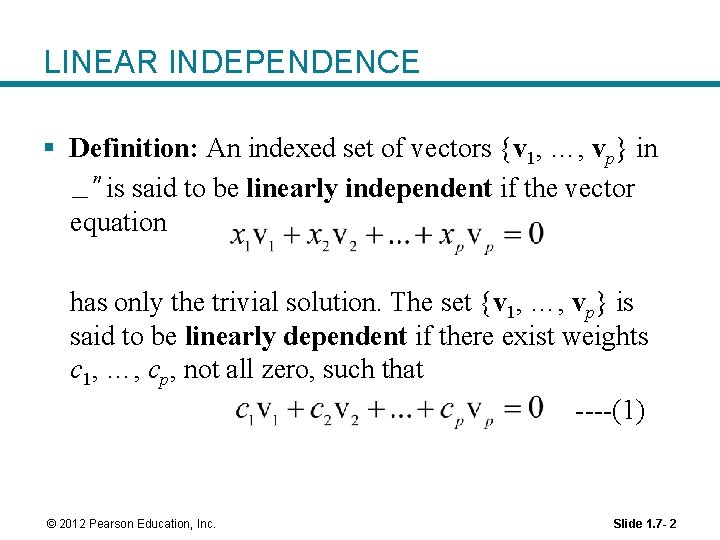LINEAR INDEPENDENCE § Definition: An indexed set of vectors {v 1, …, vp} in is said to be linearly independent if the vector equation has only the trivial solution. The set {v 1, …, vp} is said to be linearly dependent if there exist weights c 1, …, cp, not all zero, such that ----(1) © 2012 Pearson Education, Inc. Slide 1. 7 - 2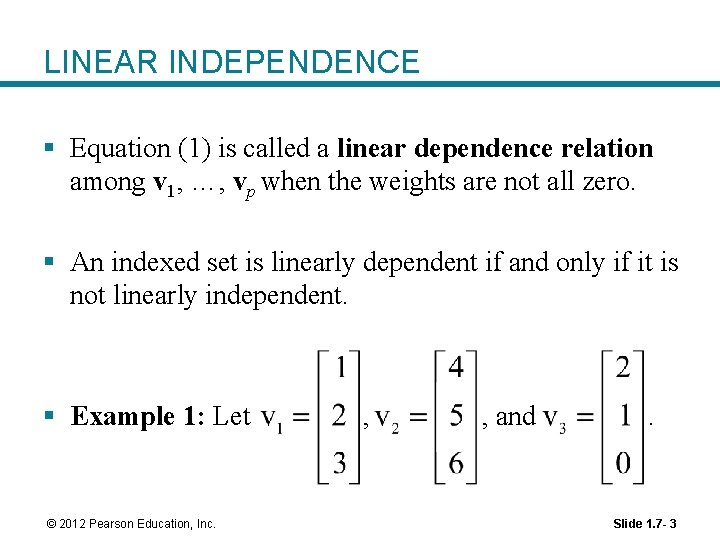LINEAR INDEPENDENCE § Equation (1) is called a linear dependence relation among v 1, …, vp when the weights are not all zero. § An indexed set is linearly dependent if and only if it is not linearly independent. § Example 1: Let © 2012 Pearson Education, Inc. , , and . Slide 1. 7 - 3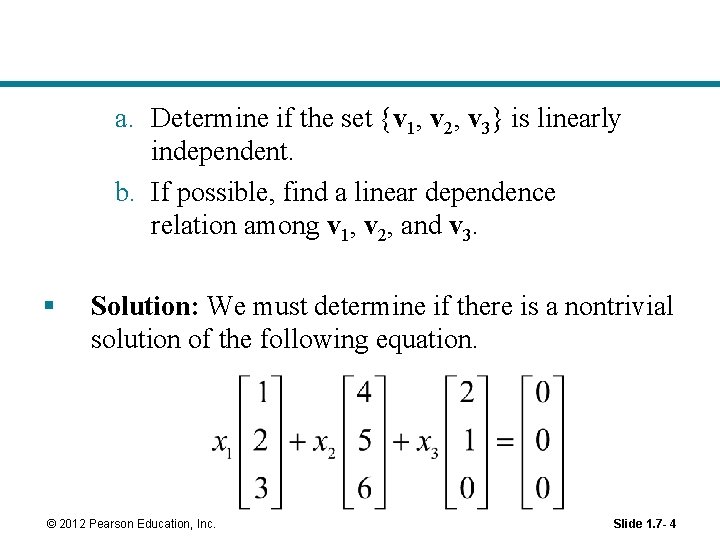a. Determine if the set {v 1, v 2, v 3} is linearly independent. b. If possible, find a linear dependence relation among v 1, v 2, and v 3. § Solution: We must determine if there is a nontrivial solution of the following equation. © 2012 Pearson Education, Inc. Slide 1. 7 - 4LINEAR INDEPENDENCE § Row operations on the associated augmented matrix show that. § x 1 and x 2 are basic variables, and x 3 is free. § Each nonzero value of x 3 determines a nontrivial solution of (1). § Hence, v 1, v 2, v 3 are linearly dependent. © 2012 Pearson Education, Inc. Slide 1. 7 - 5LINEAR INDEPENDENCE b. To find a linear dependence relation among v 1, v 2, and v 3, row reduce the augmented matrix and write the new system: § § § Thus, , , and x 3 is free. Choose any nonzero value for x 3—say, Then and. © 2012 Pearson Education, Inc. . Slide 1. 7 - 6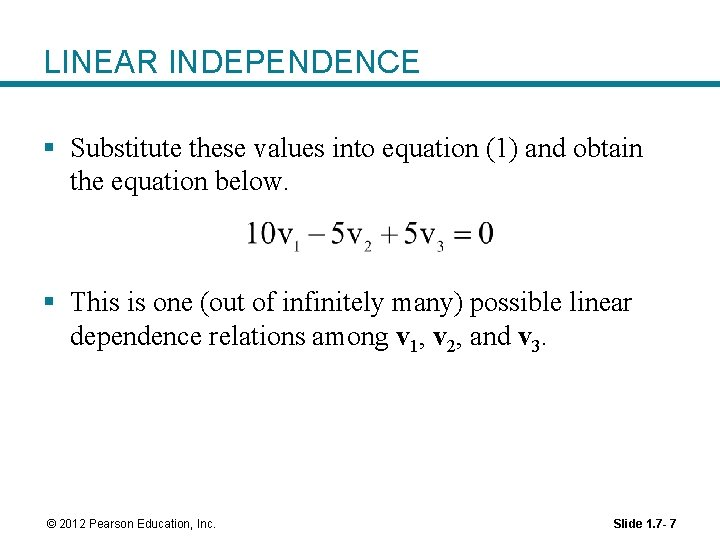LINEAR INDEPENDENCE § Substitute these values into equation (1) and obtain the equation below. § This is one (out of infinitely many) possible linear dependence relations among v 1, v 2, and v 3. © 2012 Pearson Education, Inc. Slide 1. 7 - 7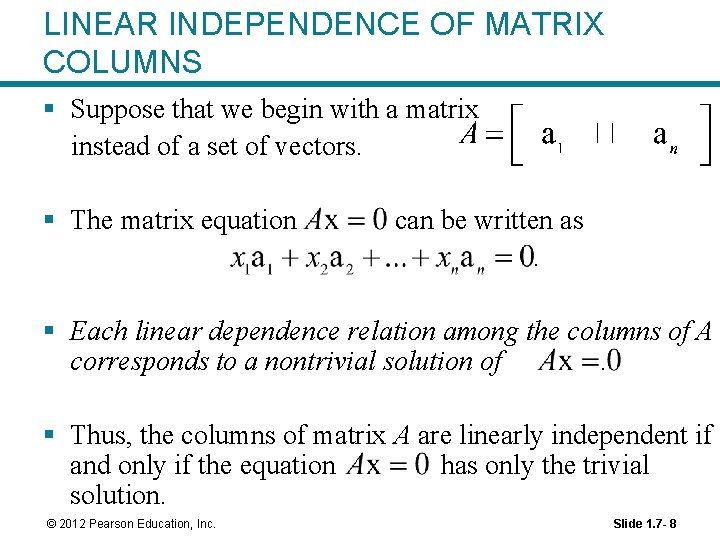LINEAR INDEPENDENCE OF MATRIX COLUMNS § Suppose that we begin with a matrix instead of a set of vectors. § The matrix equation can be written as. § Each linear dependence relation among the columns of A corresponds to a nontrivial solution of. § Thus, the columns of matrix A are linearly independent if and only if the equation has only the trivial solution. © 2012 Pearson Education, Inc. Slide 1. 7 - 8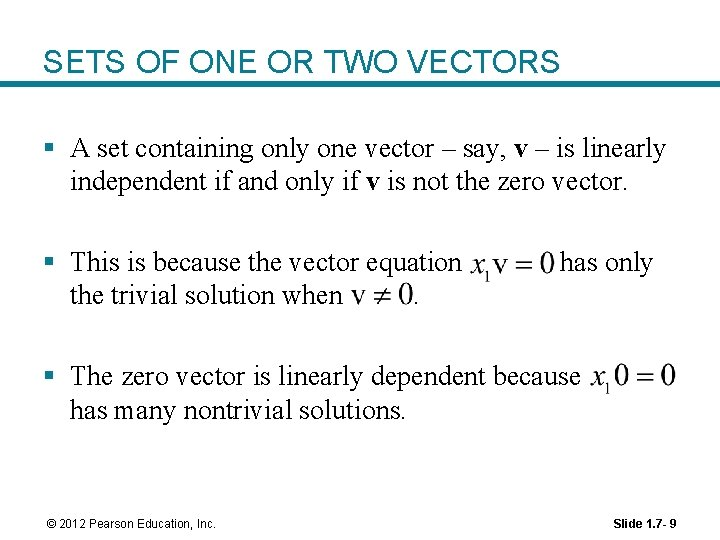SETS OF ONE OR TWO VECTORS § A set containing only one vector – say, v – is linearly independent if and only if v is not the zero vector. § This is because the vector equation the trivial solution when. has only § The zero vector is linearly dependent because has many nontrivial solutions. © 2012 Pearson Education, Inc. Slide 1. 7 - 9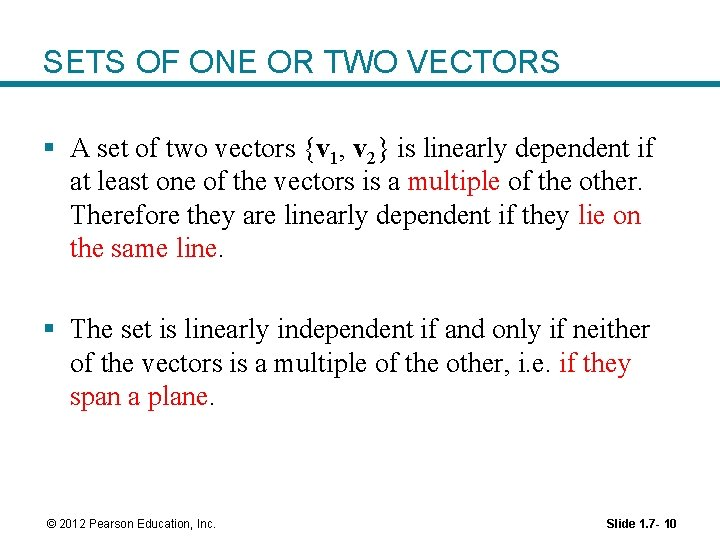SETS OF ONE OR TWO VECTORS § A set of two vectors {v 1, v 2} is linearly dependent if at least one of the vectors is a multiple of the other. Therefore they are linearly dependent if they lie on the same line. § The set is linearly independent if and only if neither of the vectors is a multiple of the other, i. e. if they span a plane. © 2012 Pearson Education, Inc. Slide 1. 7 - 10SETS OF TWO OR MORE VECTORS § Theorem 7: Characterization of Linearly Dependent Sets § An indexed set of two or more vectors is linearly dependent if and only if at least one of the vectors in S is a linear combination of the others. § In fact, if S is linearly dependent and , then some vj (with ) is a linear combination of the preceding vectors, v 1, …, . © 2012 Pearson Education, Inc. Slide 1. 7 - 11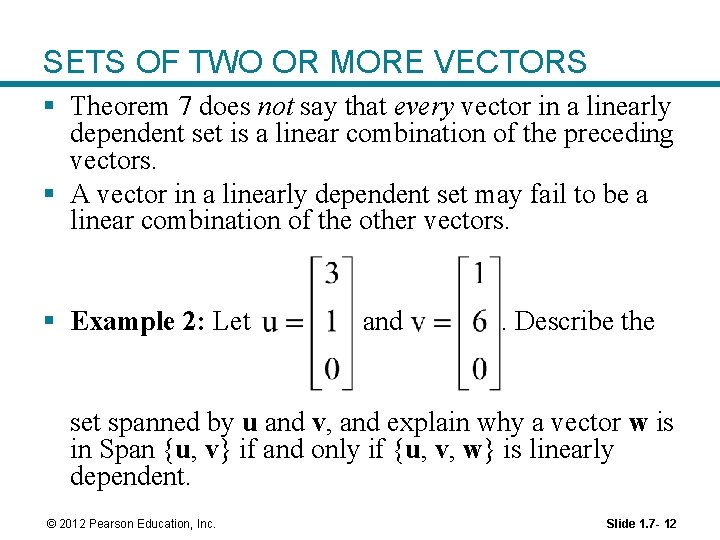SETS OF TWO OR MORE VECTORS § Theorem 7 does not say that every vector in a linearly dependent set is a linear combination of the preceding vectors. § A vector in a linearly dependent set may fail to be a linear combination of the other vectors. § Example 2: Let and . Describe the set spanned by u and v, and explain why a vector w is in Span {u, v} if and only if {u, v, w} is linearly dependent. © 2012 Pearson Education, Inc. Slide 1. 7 - 12SETS OF TWO OR MORE VECTORS § Solution: The vectors u and v are linearly independent because neither vector is a multiple of the other, and so they span a plane in. § Span {u, v} is the x 1 x 2 -plane (with ). § If w is a linear combination of u and v, then {u, v, w} is linearly dependent, by Theorem 7. § Conversely, suppose that {u, v, w} is linearly dependent. § By theorem 7, some vector in {u, v, w} is a linear combination of the preceding vectors (since ). § That vector must be w, since v is not a multiple of u. © 2012 Pearson Education, Inc. Slide 1. 7 - 13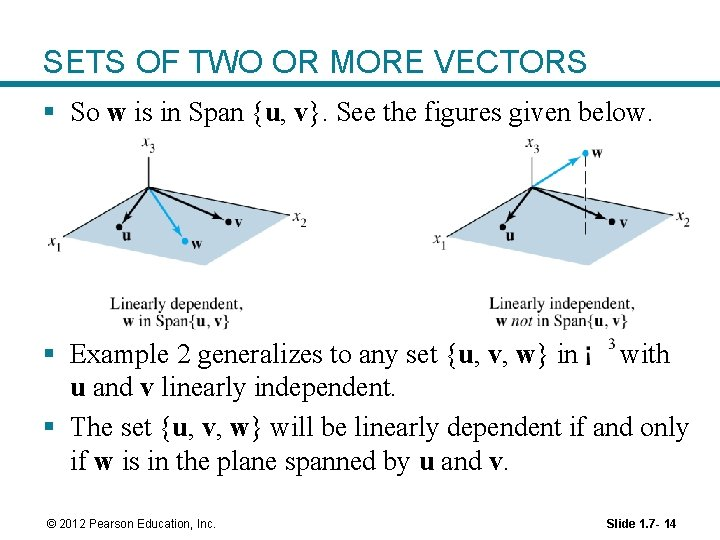SETS OF TWO OR MORE VECTORS § So w is in Span {u, v}. See the figures given below. § Example 2 generalizes to any set {u, v, w} in with u and v linearly independent. § The set {u, v, w} will be linearly dependent if and only if w is in the plane spanned by u and v. © 2012 Pearson Education, Inc. Slide 1. 7 - 14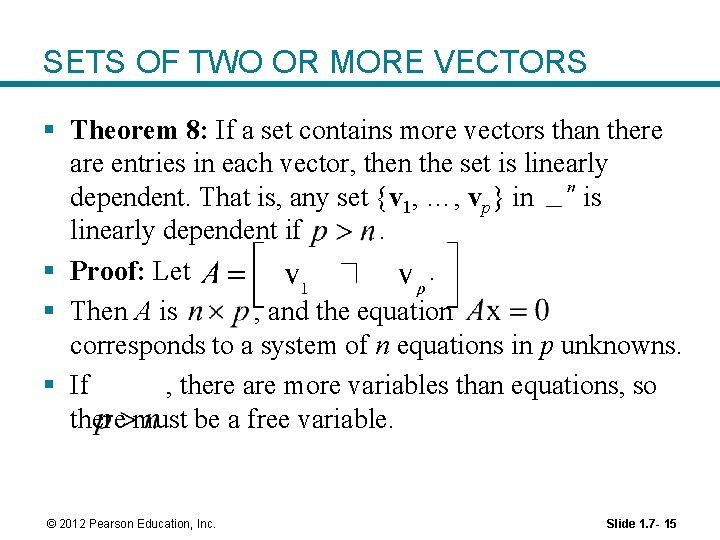SETS OF TWO OR MORE VECTORS § Theorem 8: If a set contains more vectors than there are entries in each vector, then the set is linearly dependent. That is, any set {v 1, …, vp} in is linearly dependent if. § Proof: Let. § Then A is , and the equation corresponds to a system of n equations in p unknowns. § If , there are more variables than equations, so there must be a free variable. © 2012 Pearson Education, Inc. Slide 1. 7 - 15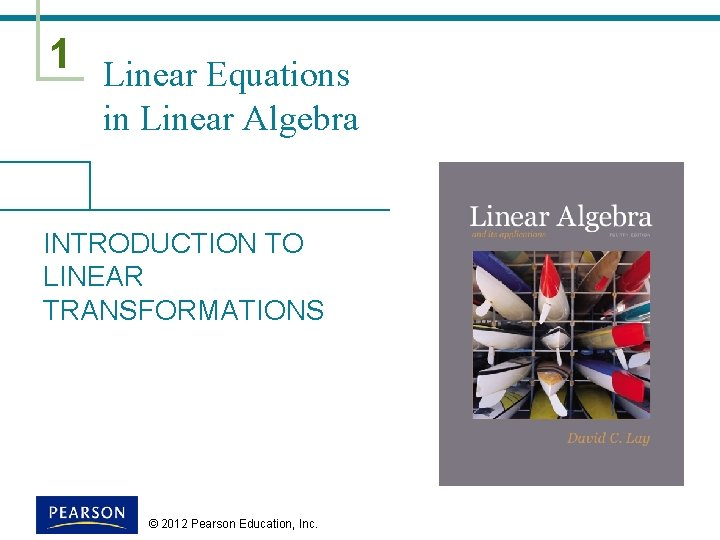1 Linear Equations in Linear Algebra INTRODUCTION TO LINEAR TRANSFORMATIONS © 2012 Pearson Education, Inc.LINEAR TRANSFORMATIONS § A transformation (or function or mapping) T from to is a rule that assigns to each vector x in a vector T (x) in. § The set is called domain of T, and is called the codomain of T. § The notation indicates that the domain of T is and the codomain is. § For x in , the vector T (x) in is called the image of x (under the action of T ). § The set of all images T (x) is called the range of T. See the figure on the next slide. © 2012 Pearson Education, Inc. Slide 1. 8 - 17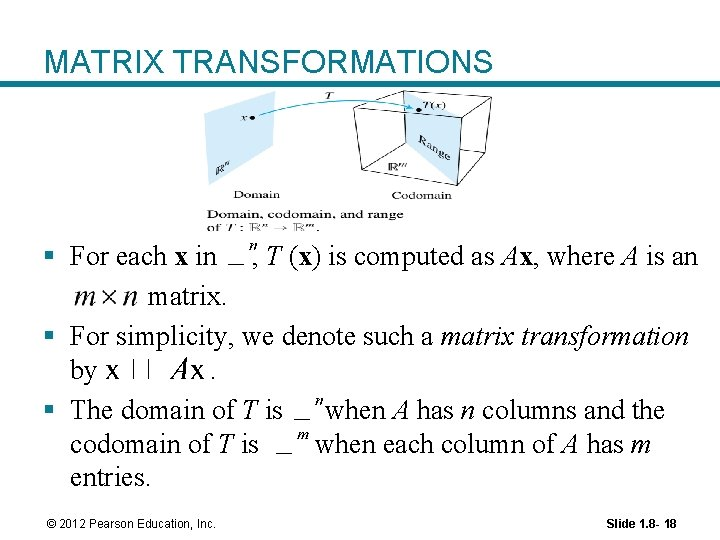MATRIX TRANSFORMATIONS § For each x in , T (x) is computed as Ax, where A is an matrix. § For simplicity, we denote such a matrix transformation by. § The domain of T is when A has n columns and the codomain of T is when each column of A has m entries. © 2012 Pearson Education, Inc. Slide 1. 8 - 18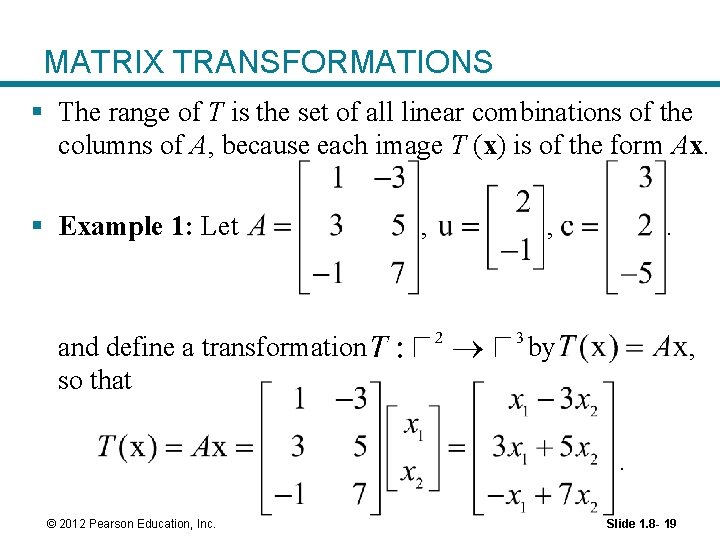MATRIX TRANSFORMATIONS § The range of T is the set of all linear combinations of the columns of A, because each image T (x) is of the form Ax. § Example 1: Let and define a transformation so that , , . by , . © 2012 Pearson Education, Inc. Slide 1. 8 - 19MATRIX TRANSFORMATIONS a. Find T (u), the image of u under the transformation T. b. Find an x in whose image under T is b. c. Is there more than one x whose image under T is b? d. Determine if c is in the range of the transformation T. © 2012 Pearson Education, Inc. Slide 1. 8 - 20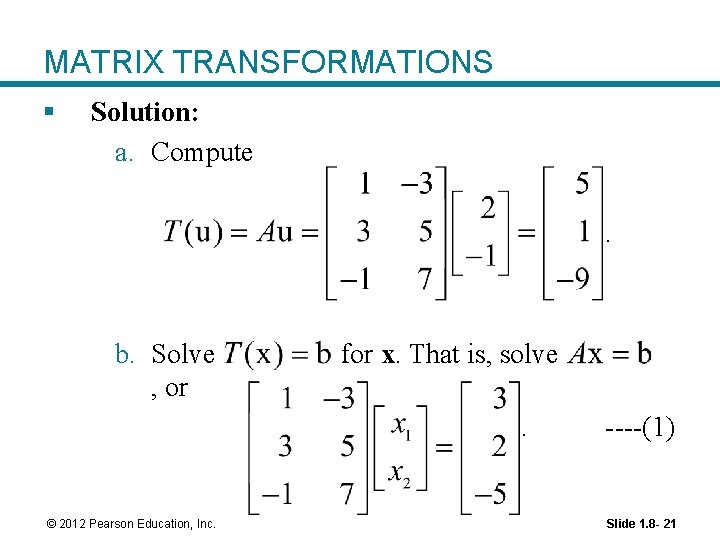MATRIX TRANSFORMATIONS § Solution: a. Compute. b. Solve , or for x. That is, solve. © 2012 Pearson Education, Inc. ----(1) Slide 1. 8 - 21MATRIX TRANSFORMATIONS § Row reduce the augmented matrix: ----(2) § Hence , , and . § The image of this x under T is the given vector b. © 2012 Pearson Education, Inc. Slide 1. 8 - 22MATRIX TRANSFORMATIONS c. Any x whose image under T is b must satisfy equation (1). § From (2), it is clear that equation (1) has a unique solution. § So there is exactly one x whose image is b. d. The vector c is in the range of T if c is the image of some x in , that is, if for some x. § This is another way of asking if the system is consistent. © 2012 Pearson Education, Inc. Slide 1. 8 - 23MATRIX TRANSFORMATIONS § To find the answer, row reduce the augmented matrix. § The third equation, system is inconsistent. , shows that the § So c is not in the range of T. © 2012 Pearson Education, Inc. Slide 1. 8 - 24SHEAR TRANSFORMATION § Example 2: Let defined by transformation. . The transformation is called a shear § It can be shown that if T acts on each point in the square shown in the figure on the next slide, then the set of images forms the shaded parallelogram. © 2012 Pearson Education, Inc. Slide 1. 8 - 25SHEAR TRANSFORMATION § The key idea is to show that T maps line segments onto line segments and then to check that the corners of the square map onto the vertices of the parallelogram. § For instance, the image of the point is , © 2012 Pearson Education, Inc. Slide 1. 8 - 26LINEAR TRANSFORMATIONS and the image of § § is . T deforms the square as if the top of the square were pushed to the right while the base is held fixed. Definition: A transformation (or mapping) T is linear if: i. for all u, v in the domain of T; ii. for all scalars c and all u in the domain of T. © 2012 Pearson Education, Inc. Slide 1. 8 - 27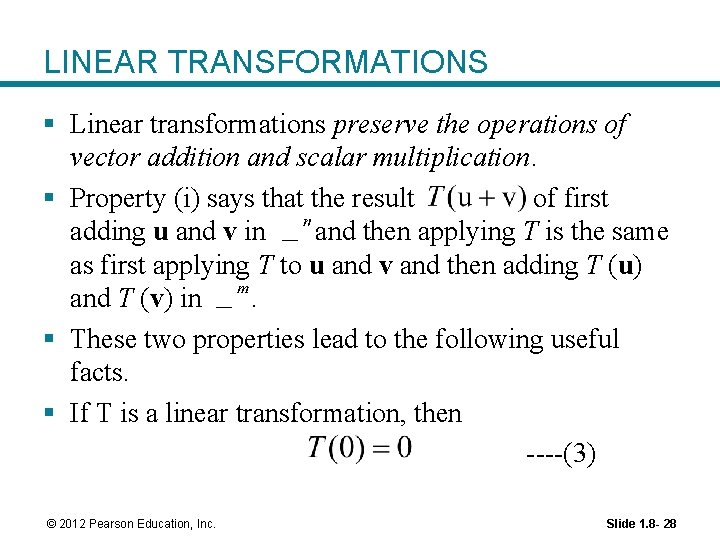LINEAR TRANSFORMATIONS § Linear transformations preserve the operations of vector addition and scalar multiplication. § Property (i) says that the result of first adding u and v in and then applying T is the same as first applying T to u and v and then adding T (u) and T (v) in. § These two properties lead to the following useful facts. § If T is a linear transformation, then ----(3) © 2012 Pearson Education, Inc. Slide 1. 8 - 28LINEAR TRANSFORMATIONS and. ----(4) for all vectors u, v in the domain of T and all scalars c, d. § Property (3) follows from condition (ii) in the definition, because. § Property (4) requires both (i) and (ii): § If a transformation satisfies (4) for all u, v and c, d, it must be linear. § (Set for preservation of addition, and set for preservation of scalar multiplication. ) © 2012 Pearson Education, Inc. Slide 1. 8 - 29LINEAR TRANSFORMATIONS § Repeated application of (4) produces a useful generalization: ----(5) § In engineering and physics, (5) is referred to as a superposition principle. § Think of v 1, …, vp as signals that go into a system and T (v 1), …, T (vp) as the responses of that system to the signals. © 2012 Pearson Education, Inc. Slide 1. 8 - 30LINEAR TRANSFORMATIONS § The system satisfies the superposition principle if whenever an input is expressed as a linear combination of such signals, the system’s response is the same linear combination of the responses to the individual signals. § Given a scalar r, define § T is called a contraction when dilation when. © 2012 Pearson Education, Inc. by . and a Slide 1. 8 - 31Rotations § A linear transformation of the form is called a rotation by the angle © 2012 Pearson Education, Inc. Slide 1. 4 - 32